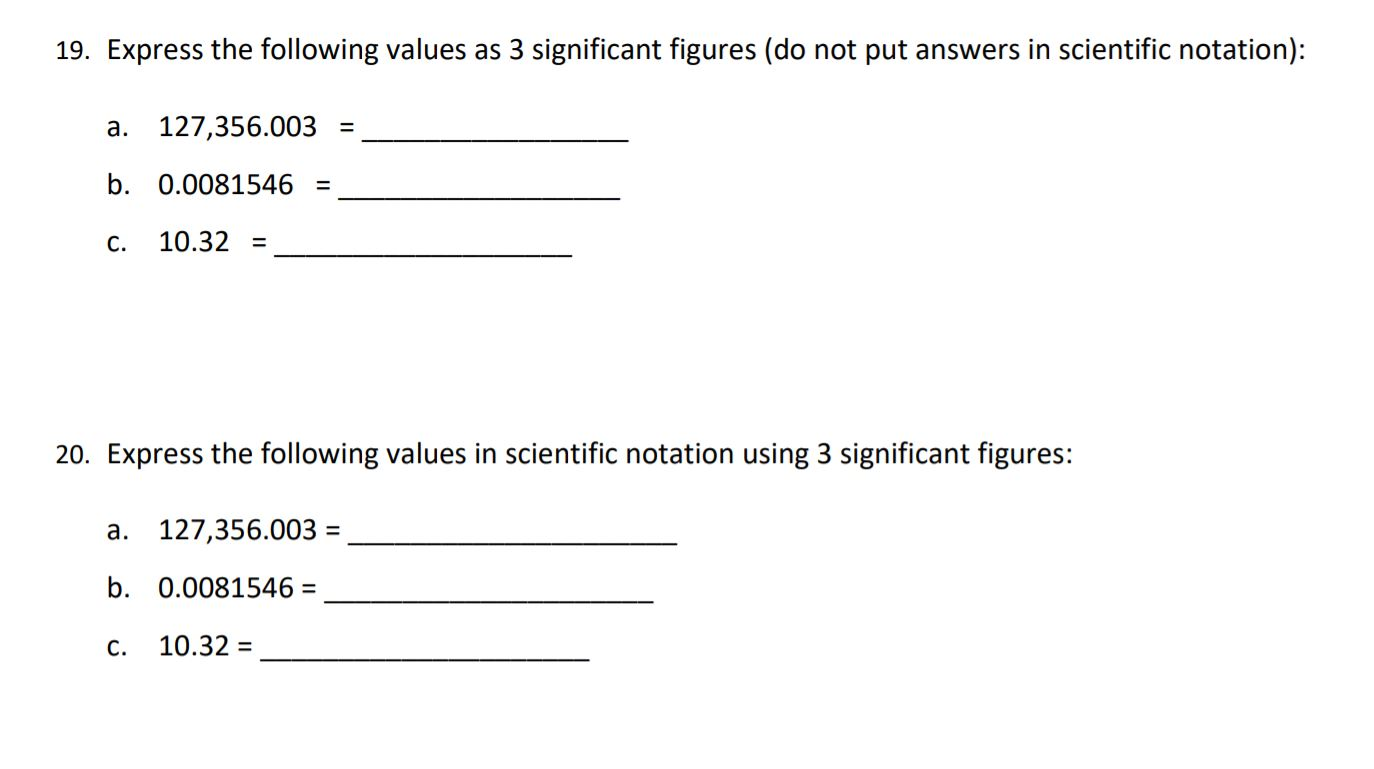### Create an Account

Home / Questions / Express the following values as 3 significant figures (do not put answers in scientific no...

# Express the following values as 3 significant figures (do not put answers in scientific notation): a. 127,356.003 = b. 0.0081546 = C. 10.32 = 20. Express the following values in scientific notation

Express the following values as 3 significant figures (do not put answers in scientific notation): a. 127,356.003 = b. 0.0081546 = C. 10.32 = 20. Express the following values in scientific notation using 3 significant figures: a. 127,356.003 = b. 0.0081546 = C. 10.32Jun 17 2021 View more View LessSubscribe To Get Solution